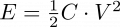# Capacitance and work, translating to battery comparisons - EE question

#### LikeTheSandwich

Joined Feb 22, 2021
164
OK, so I've been looking into the possibility of using some super caps in some personal projects instead of batteries, just for fun. In my research, it seems that farads is a measure of electrical charge, and to convert to Wh or Joules, the voltage has to be squared. I've found online calculators to input voltage and farad rating and get a Joules output to get a "total amount of energy stored" comparison. So because voltage is squared, when I'm using capacitors in series, I have to calculate the Joules of each capacitor and add them together, right? So two capacitors in series doesn't somehow magically create four times the amount of energy that can be stored, right? Or due to the function of capacitors, does it actually do that somehow?

#### ApacheKid

Joined Jan 12, 2015
1,271
OK, so I've been looking into the possibility of using some super caps in some personal projects instead of batteries, just for fun. In my research, it seems that farads is a measure of electrical charge, and to convert to Wh or Joules, the voltage has to be squared. I've found online calculators to input voltage and farad rating and get a Joules output to get a "total amount of energy stored" comparison. So because voltage is squared, when I'm using capacitors in series, I have to calculate the Joules of each capacitor and add them together, right? So two capacitors in series doesn't somehow magically create four times the amount of energy that can be stored, right? Or due to the function of capacitors, does it actually do that somehow?
Farad's are not a measure of charge (Q) but of "capacitance" (C).

A given capacitor with a given voltage across it, contains charge C * V.

The energy E is (as I'm sure you know):Adjusting C by adding/removing capacitors in series of parallel does not change the equation, that law does not change.

If you had two circuits with 10 v across it and one had a 100 uF capacitor and the other had two 200 uF capacitors in series, the stored energy would be the same.

#### LikeTheSandwich

Joined Feb 22, 2021
164
So a 10V, 100F cap stores 5000J, right? So if I put two in series, and charge them to 10V each, making 20V across the pair and 200F total capacitance, is the equation this: 200F/2 * 20V^2 = 40,000J? So total energy goes up by 8x somehow? Or do I need to calculate each capacitor individually, and then add the results like with batteries?

#### ApacheKid

Joined Jan 12, 2015
1,271
Yes, 5,000 J.

No, if you put capacitors in series you reduce the capacitance, unlike resistors.

So two 100 F in series is the same as one 50 F.

Putting 20V across that yields 10,000 J.

If you put them in parallel and also apply 20 V you'll store 40,000 J

#### BobTPH

Joined Jun 5, 2013
8,069
Two 100F capacitors in series makes a 50F capacitor. So you have either a 20V 50F cap or two 100F 10V caps and the energy comes out the same.

Bob

#### LikeTheSandwich

Joined Feb 22, 2021
164
OK, that makes sense with the equation. Interesting. I wonder why that is.

#### ApacheKid

Joined Jan 12, 2015
1,271
OK, that makes sense with the equation. Interesting. I wonder why that is.
Its the surface area, two 1 inch square plates add up to 2 square inches. Increasing surface are increases capacitance

#### LikeTheSandwich

Joined Feb 22, 2021
164
Yes, that part is intuitive. But caps in series reducing capacitance is counter-intuitive. I wonder why that happens.

#### ApacheKid

Joined Jan 12, 2015
1,271
Yes, that part is intuitive. But caps in series reducing capacitance is counter-intuitive. I wonder why that happens.
Similar thing, but this time distance, distance between the plates is doubled - effectively.

C depends on both area and distance between the "plates".

#### LikeTheSandwich

Joined Feb 22, 2021
164
Ah, that is indeed intuitive actually, when put into those terms. Thank you.

#### ApacheKid

Joined Jan 12, 2015
1,271
I used to know this inside out, but I got rusty working with software for so long, so I've been reviewing all this basic theory recently.

•LikeTheSandwich